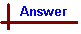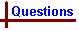CHEMICAL FORMULAS AND NOMENCLATURE (Questions) Your feedback on these self-help problems is appreciated. Click here to send an e-mail. Shortcut to Questions Q: 1 2 3 4 5 6 7 8 9 10 11 12 13 1 Write systematic names for each of the following binary compounds. (1.) CO (2.) HBr (3.) PBr3 (4.) N2O32 Write systematic names for each of the following binary compounds. (1.) CCl4 (2.) NaH (3.) OF2 (4.) H2O2 (5.) NO23 Write correct formulas for each of the following binary compounds. (1.) lead(IV) oxide (2.) copper(I) chloride (3.) rubidium peroxide (4.) magnesium nitride (5.) calcium hydride4 Write correct formulas for each of the following binary compounds. (1.) ammonia (2.) phosphine (3.) silane (4.) tin tetrachloride (5.) phosphorus pentoxide5 The following series of hydroxo acids containing chlorine exists. Give them their systematic names. (1.) HClO or (HOCl) (2.) HClO2 or (HOClO) (3.) HClO3 or (HOClO2) (4.) HClO4 or (HOClO3)6 Phosphoric acid H3PO4 (or (HO)3PO) is a triprotic acid. Name the following species. (1.) H2PO4- (2.) HPO42- (3.) PO43-7 Give systematic names for the following species. (1.) H2SO4 (2.) NaHSO4 (3.) Na2SO4 (4.) H2SO3 (5.) LiHSO3 (6.) Li2SO38 Give systematic names for the following species. (1.) HNO3 (2.) HNO2 (3.) Ca(NO2)2 (4.) H2CO3 (5.) KHCO3 (6.) BaCl2 (7.) Co3(PO4)2 (8.) Hg2Cl2 (9.) HgCl29 Give systematic names for the following complexes: (1.) [Ag(NH3)2]+ (2.) [AgCl2]- (3.) [Pb(OH)4]2- (4.) [Al(H2O)6]3+ (5.) [Al(OH)4]- (6.) [Co(NH3)6]3+10 Give systematic names for the following complexes: (1.) [CoCl6]3- (2.) [CoCl2(NH3)4]+ (3.) [PdCl2(NH3)2] (4.) [HgI4]2- (5.) [BF4]- (6.) [AlH4]- (7.) [CrCl2(NH3)2(H2O)2][BF4]11 Write formulas for the following substances. (1.) ammonium chloride (2.) copper(II) sulfate-5-water (3.) manganese(II) sulfide (4.) barium chromate (5.) sodium dichromate12 Write formulas for the following substances. (1.) strontium hydrogensulfate (2.) sodium hydrogencarbonate (3.) aluminium potassium sulfate-12-water (4.) ammonium iron(II) sulfate-6-water (5.) sodium dihydrogenphosphate13 Write formulas for the following substances. (1.) potassium hexacyanoferrate(III) (2.) sodium hexacyanoferrate(II) (3.) tetraamminedichlorocobalt(III) chloride (4.) diamminesilver(I) chloride (5.) lithium tetrahydridoaluminateCHEMICAL FORMULAS AND NOMENCLATURE (Answers) 1 (1.) carbon monoxide (2.) hydrogen bromide (3.) phosphorus tribromide (or phosphorus(III) bromide) (4.) dinitrogen trioxide (or nitrogen(III) oxide) Note that prefixes designating how many atoms of each element are present in a molecule are only used when more than one combination is possible. For example, phosphorus can form the bromides PBr3 or PBr5 (depending on its oxidation state), so the tri- prefix is used to specify PBr3 and avoid any ambiguity. Hydrogen only forms one bromide (HBr), so no prefix is used. Oxidation states can be specified in compound names instead of prefixes, so dinitrogen trioxide could also be called nitrogen(III) oxide, although the prefix system is usually prefered for covalent compounds.2 (1.) carbon tetrachloride (2.) sodium hydride (3.) oxygen fluoride (4.) hydrogen peroxide (5.) nitrogen dioxide   Note that OF2 is oxygen fluoride and not fluorine oxide, since fluorine is the more electronegative element. Similarly, inNO2, oxygen is more electronegative than nitrogen.3 (1.) PbO2 (2.) CuCl (3.) Rb2O2 (4.) Mg3N2 (5.) CaH2   (1.) and (2.) are examples of an element's oxidation states determining the formula of the compound. Note that oxidation states are only explicitly written in the compound name when more than one oxidation state is possible.4 (1.) NH3 (2.) PH3 (3.) SiH4 (4.) SnCl4 (5.) P2O5   Parts (1.) - (3.) are examples of hydrides with special names. These names are always used for these compounds (ie NH3 is always called ammonia, never nitrogen hydride). Note that P2O5 is not referred to as diphosphorus pentoxide, since both possible forms of phosphorus oxide (P2O3 and P2O5) contain two phosphorus atoms in their formula.5 (1.) hypochlorous acid (2.) chlorous acid (3.) chloric acid (4.) perchloric acid Note the change in nomenclature with varying oxidation state of the chlorine in this series of acids.6 (1.) dihydrogenphosphate ion (2.) hydrogenphosphate ion or monohydrogenphosphate (3.) phosphate ion   Hydrogenphosphate is sometimes referred to as monohydrogenphosphate, to emphasise the presence of only one hydrogen in the molecule. Note also that these names are written as one word, with no space between the individual terms.7 (1.) sulfuric acid (2.) sodium hydrogensulfate (3.) sodium sulfate (4.) sulfurous acid (5.) lithium hydrogensulfite (6.) lithium sulfite   Note the use of hydrogensulfate in (2.), since this is the only acid sulfate sodium can form.8 (1.) nitric acid (2.) nitrous acid (3.) calcium nitrite (4.) carbonic acid (5.) potassium hydrogencarbonate (6.) barium chloride (7.) cobalt(II) phosphate (8.) mercury(I) chloride (9.) mercury(II) chloride   Compounds (7.) to (9.) involve metals which can exist in various oxidation states, so the oxidation states are explicitly written in the name. Note that mercury(I) compounds always contain the Hg22+ ion, hence the formula Hg2Cl2 for mercury(I) chloride, and not HgCl.9 (1.) diamminesilver(I) ion (not diaminosilver or diammoniasilver ion) (2.) dichloroargentate(I) ion (not dichlorosilvate(I) ion) (3.) tetrahydroxoplumbate(II) ion (not tetrahydroxoleadate ion) (4.) hexaaquaaluminium ion (not hexawateraluminium ion) (5.) tetrahydroxoaluminate ion (not tetrahydroxoaluminium ion) (6.) hexaamminecobalt(III) ion (note double vowels are written as double aa)   Square brackets indicate a complex species which require use of the appropriate nomenclature. If the complex is positive or neutral, the central metal is written with its proper name (eg. diamminesilver ion in (1.)), wheras the central metal atom of negatively charged complexes is given the suffix -ate (eg. tetrahydroxoaluminate ion in (5.)). In addition, some metals have latin names which are used to name them in negatively charged complexes (eg. dichloroargentate ion in (2.)). Note the designation of oxidation states in (1), (2.), (3.) and (6.) since silver, lead and cobalt may exist in various oxidation states, which affect the overall stoichiometry of the compound. The ligands H2O and NH3 have special names, aqua and ammine, respectively. Negatively charged ligands always end in "o" eg OH- is hydroxo not hydroxy.10 (1.) hexachlorocobaltate(III) ion (2.) tetraamminedichlorocobalt(III) ion (3.) diamminedichloropalladium(II) (4.) tetraiodomercurate(II) ion (5.) tetrafluoroborate ion (6.) tetrahydridoaluminate ion (7.) diamminediaquadichlorochromium(III) tetrafluoroborate   Note the use of cobaltate in the negatively charged complex (1.), and cobalt in the positively charged complex (2.). Also note the alphabetical order of ligand names within complexes (2.), (3.) and (7.) (for example (3.) is diamminedichloropalladium(II), not dichlorodiamminepalladium(II)).11 (1.) NH4Cl (2.) CuSO4.5H2O (3.) MnS (4.) BaCrO4 (5.) Na2Cr2O7    Note that manganese can exist in a number of oxidation states. Also note the difference in formula between chromate ion (CrO42- in (4.)) and dichromate ion (Cr2O72- in (5.)).12 (1.) Sr(HSO4)2 (2.) NaHCO3 (3.) KAl(SO4)2.12H2O (4.) (NH4)2Fe(SO4)2.6H2O (5.) NaH2PO4 (3.) and (4.) are examples of double salts containing more than one species of positive ion, written with positive ions followed by negative ions, and then water of crystallisation (if present).13 (1.) K3[Fe(CN)6] (2.) Na4[Fe(CN)6] (3.) [CoCl2(NH3)4]Cl (4.) [Ag(NH3)2]Cl (5.) Li[AlH4] The differences in formulae between (1.) and (2.) stem from the differences in oxidation states of the Fe atoms in the hexacyanoferrate complex. These determine the charge of the complex, and hence the stoichiometry of the formula.